Courses

# Mechanics of Rotational Motion: JEE Main (Part- 2) - Physics, Solution by DC Pandey NEET Notes | EduRev

## DC Pandey (Questions & Solutions) of Physics: NEET

Created by: Ciel Knowledge

## NEET : Mechanics of Rotational Motion: JEE Main (Part- 2) - Physics, Solution by DC Pandey NEET Notes | EduRev

The document Mechanics of Rotational Motion: JEE Main (Part- 2) - Physics, Solution by DC Pandey NEET Notes | EduRev is a part of the NEET Course DC Pandey (Questions & Solutions) of Physics: NEET.
All you need of NEET at this link: NEET

Objective Questions
Single Correct Option

Ques 1: The moment of inertia of a body does not depend on
(a) mass of the body
(b) the distribution of the mass in the body
(c) the axis of rotation of the body
(d) None of these
Ans: d
Solution: I = Mk2, which depends upon, mass and its distribution about an axis

Ques 2: The radius of gyration of a disc of radius 25 cm is
(a) 18 cm
(b) 12.5 cm
(c) 36 cm
(d) 50 cm
Ans: (a)
Solution: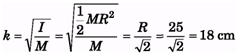Ques 3: A shaft initially rotating at 1725 rpm is brought to rest uniformly in 20s. The number of revolutions that the shaft will make during this time is        (a) 1680
(b) 575
(c) 287
(d) 627
Ans: (c)
Solution: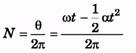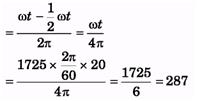Ques 4: A man standing on a platform holds weights in his outstretched arms. The system is rotated about a central vertical axis. If the man now pulls the weights inwards close to his body, then
(a) the angular velocity of the system will increase
(b) the angular momentum of the system will remain constant
(c) the kinetic energy of the system will increase
(d) All of the above
Ans: (d)
Solution: In pulling hands closes, external torque is zero, so angular momentum remains constant. Due to decrease in moment of inertia, angular velocity increases and KE increases.

Ques 5: The moment of inertia of a uniform semicircular disc of mass M and radius rabout a line perpendicular to the plane of the disc through the centre is
(a) Mr2
(b) 1/2Mr2
(c) 1/4Mr2
(d)2/5Mr2

Ans: (b)

Ques 6: Two bodies A and B made of same material have the moment of inertial in the ratio IA : IB = 16 : 18. The ratio of the masses mA : mB is given by  (a) cannot be obtained
(b) 2:3
(c) 1 : 1
(d) 4 : 9
Ans: (a)
Solution: Masses cannot be compared as distribution is not given.

Ques 7: When a sphere rolls down an inclined plane, then identity the correct statement related to the work done by friction force
(a) The friction force does positive translational work
(b) The friction force does negative rotational work
(c) The net work done by friction is zero
(d) All of the above
Ans: (c)
Solution:As the displacement of point of contact is zero, so work done by friction is zero.

Ques 8: A circular table rotates about a vertical axis with a constant angular speed w. A circular pan rests on the turn table (with the centre coinciding with centre of table) and rotates with the table. The bottom of the pan is covered with a uniform thick layer of ice which also rotates with the pan. The ice starts melting. The angular speed of the turn table
(a) remains the same
(b) decreases
(c) increases
(d) may increase or decrease depending on the thickness of ice layer
Ans: (b)
Solution: Due to melting of ice, water spreads on outer side of the pan, increasing moment of inertia, which leads to a decrease in angular speed due to conservation of angular momentum

Ques 9: If R is the radius of gyration of a body of mass M and radius r, then the ratio of its rotational to translational kinetic energy in the rolling condition is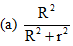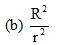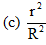(d) 1

Ans: (b)
Solution: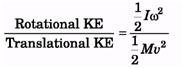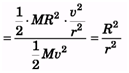Ques 10: A solid sphere rolls down two different inclined planes of the same height but of different inclinations
(a) in both cases the speeds and time of descend will be same
(b) the speeds will be same but time of descend will be different
(c) the speeds will be different but time of descend will be same
(d) speeds and time of descend both will be different
Ans: (b)
Solution: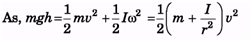⇒ v = constant, irrespective of the inclination, but time is different because of different acceleration due to different inclination

Ques 11: For the same total mass which of the following will have the largest moment of inertia about an axis passing through the centre of mass and perpendicular to the plane of the body
(a) a disc of radius R
(b) a ring of radius R
(c) a square lamina of side 2R
(d) four rods forming a square of side 2i?
Ans: (d)
Solution
: Moment of inertia is maximum, when masses are at maximum distance from axis.

Ques 12: A disc and a solid sphere of same mass and radius roll down an inclined plane. The ratio of the friction force acting on the disc and sphere is

(a)7/6
(b) 5/4
(c) 3/2
(d) depends on angle of inclination

Ans: (a)
Solution: mg sin q - f = ma and fR =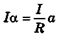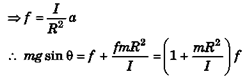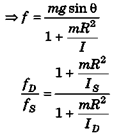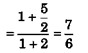Ques 13: A horizontal disc rotates freely with angular velocity w about a vertical axes through its centre. A ring, having the same mass and radius as the disc, is now gently placed coaxially on the disc. After some time, the two rotate with a common angular velocity. Then

(a) no friction exists between the disc and the ring
(b) the angular momentum of the system is conserved
(c) the final common angular velocity is 1/2ω
(d) All of the above
Ans: (b)
solution: As, there is no external torque, so angular momentum is conserved

Ques 14: A solid homogeneous sphere is moving on a rough horizontal surface, partly rolling and partly sliding. During this kind of motion of the sphere
(a) total kinetic energy of the sphere is conserved
(b) angular momentum of the sphere about any point on the horizontal surface is conserved
(c) only the rotational kinetic energy about the centre of mass is conserved
(d) None of the above
Ans: (b)
Solution: As torque due to friction about any point on horizontal surface is zero, so angular moment about that point is conserved

Ques 15: A particle of mass m = 3 kg moves along a straight line 4y - 3x = 2 where x and y are in metre, with constant velocity v =5ms-1. The magnitude of angular momentum about the origin is
(a) 12 kg m2s-1
(b) 6.0 kg m2s-1
(c) 4.5 kg m2s-1
(d) 8.0 kg m2s-1
Ans: (b)
Sol: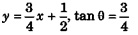⇒ θ = 37° ⇒ cos θ 4/5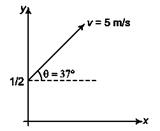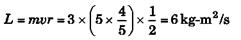Ques 16: A solid sphere rolls without slipping on a rough horizontal floor, moving with a speed v. It makes an elastic collision with a smooth vertical wall. After impact,
(a) it will move with a speed v initially
(b) its motion will be rolling with slipping initially and its rotational motion will stop momentarily at some instant
(c) its motion will be rolling without slipping only after some time
(d) All of the above
Ans: (d)
Solution:  Due to elastic collision, velocity will reverse and not angular velocity, so it will take some time to again settle to uniform rolling. During this time there will be sliping and its rotation will stop for a moment.

Ques 17: The figure shows a square plate of uniform mass distribution. AA' and BB' are the two axes lying in the plane of the plate and passing through its centre of mass. It Io is the moment of inertia of the plate about A A' then its moment of inertia about the BB' axis is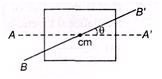(a) I0
(b) I0 cos θ
(c) Icosθ
(d) None of these

Ans: (a)
Solution: Moment of inertia about an axis perpendicular to the plane and passing through centre of a square plate is Ma2/6 and about an axis passing through its plane is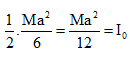is same for AA' and BB'.

Ques 18: A spool is pulled horizontally on rough surface by two equal and opposite forces as shown in the figure. Which of the following statements are correct?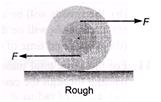(a) The centre of mass moves towards left
(b) The centre of mass moves towards right
(c) The centre of mass remains stationary
(d) The net torque about the centre of mass of the spool is zero
Ans: (b)
Solution: Due to clockwise torque, the spool will move rightward and so, will the centre of mass

Ques 19: Two identical discs are positioned on a vertical axis as shown in the figure. The bottom disc is rotating at angular velocity ω0 and has rotational kinetic energy Ko. The top disc is initially at rest.

It then falls and sticks to the bottom disc. The change in the rotational kinetic energy of the system is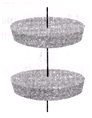(a) K0/2
(b) -K0/2
(c) -K0/4
(d) K0/4
Ans: (b)
Sol: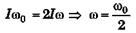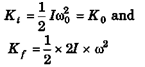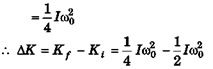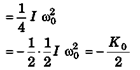Ques 20: The moment of inertia of hollow sphere (mass M) of inner radius R and outer radius 2R, having material of uniform density, about a diametric axis is
(a) 31 MR2/70
(b) 43 MR2/90
(c) 19 MR2/ 80
(d) None of these
Ans: (d)
Solution: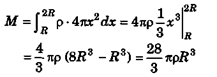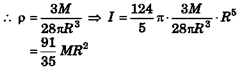Ques 21: A rod of uniform cross-section of mass M and length I is hinged about an end to swing freely in a vertical plane. However, its density is non uniform and varies linearly from hinged end to the free end doubling its value. The moment of inertia of the rod, about the rotation axis passing through the hinge point is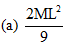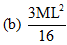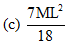(d) None of these
Ans: (c)
Solution: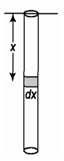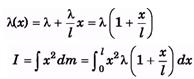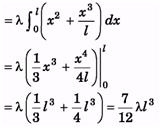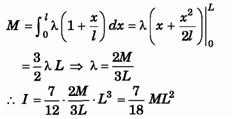Ques 22: Let I1 and I2 be the moment of inertia of a uniform square plate about axes shown in the figure. Then the ratio I1 : I2 is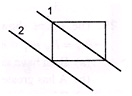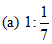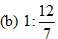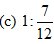(d) 1 : 7

Ans: (d)
Solution: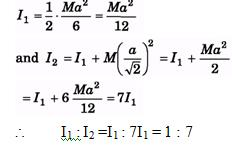Ques 23: Moment of inertia of a uniform rod of length L and mass M, about an axis passing through L/ 4 from one end and perpendicular to its length is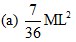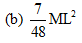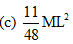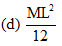Ans: (b)
Solution: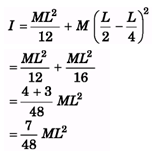Ques 24: A uniform rod of length L is free to rotate in a vertical plane about a fixed horizontal axis through B. The rod begins rotating from rest. The angular velocity ω at angle θ is given as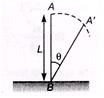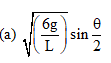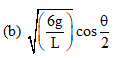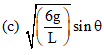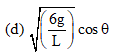Ans: (a)
Solution: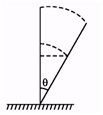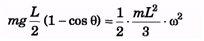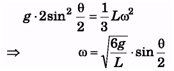Ques 25: Two particles of masses 1 kg and 2 kg are placed at a distance at a distance of 3m. Moment of inertia of the particles about an axis passing through their centre of mass and perpendicular to the line joining them is (in kg-m2).
(a) 6
(b) 9
(c) 8
(d) 12
Ans: (a)
Solution: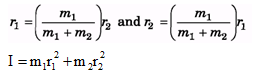Substituting the values we get,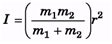Ques 26: Find moment of inertia of a thin sheet of mass M in the shape of an equilateral triangle about an axis as shown in figure. The length of each side is L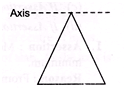(a) ML2/8
(b) 3ML2/8
(c) 7ML2/8
(d) None of these
Ans: (b)
Solution: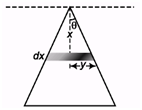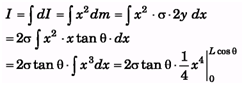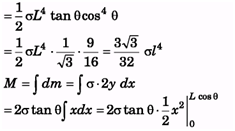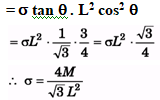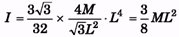Ques 27: A square is made by joining four rods each of mass M and length L. Its moment of inertia about an axis PQ, in its plane and passing through one of its corner is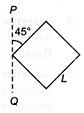(a) 6 ML2
(b)  4/3ML2
(c) 8/3ML2
(d) 10/3ML2

Ans: (c)
Solution: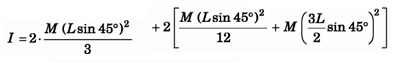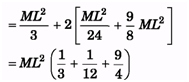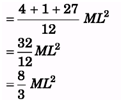Ques 28: A thin rod of length 4l, mass 4 m is bent at the points as shown in the figure. What is the moment of inertia of the rod about the axis passing through O and perpendicular to the plane of the paper?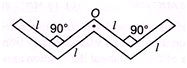(a) ml2/3
(b) 10ml2/3
(c) ml2/12
(d) ml2/24

Ans: (b)
Solution: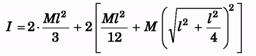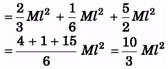Ques 29: The figure shows two cones A and B with the conditions : h< hB; rA > rB; RA = RB ; mA mB. Identify the correct statement about their axis of symmetry.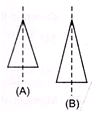(a) Both have same moment of inertia
(b) A has greater moment of inertia
(c) B has greater moment of inertia
(d) Nothing can be said
Ans: (a)
Solution: Is both have same mass and same radius, i.e., same distribution of mass, that is why both of them have same moment of inertia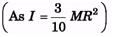Ques 30: Linear mass density of the two rods system, AC and CB is x. Moment of inertia of two rods about an axis passing through AB is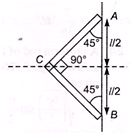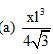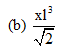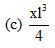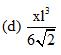Ans: (d)
Solution: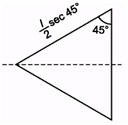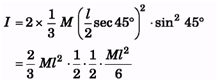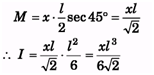Offer running on EduRev: Apply code STAYHOME200 to get INR 200 off on our premium plan EduRev Infinity!

210 docs

,

,

,

,

,

,

,

,

,

,

,

,

,

,

,

,

,

,

,

,

,

,

,

,

;